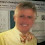## Sunday, May 20, 2018

### COMPUTER PROOF vs computer proof- Quadratic VDW theorem

Quad VDW Theorem: For all c there exists W=W(c) such that for all c-colorings of {1,...,W} there exists a,d such that a and a+d2 are the same color.

The first proof of Quad VDW was nonconstructive.

The second proof was constructive but used VDW's theorem and gave terrible bounds, even for W(2).

EASY: Show W(2)=5

ON A HS MATH COMP: Show that for all 3-colorings of {1,...,2003} there exists two numbers a square apart that are the same color.

One can get a bound less than 100.

With a lot more detailed work one can get W(3)=29.

None of above was done with a computer.

SO- what about W(4)?  There are three proofs that W(4) exists:

a) Prove the QUAD VDW theorem. This gives terrible bounds.

b) I had some students use SAT solvers and they obtained W(4)=58. I am sure they are right and I am glad to know it (especially since it confirms the general mantra that the bounds from proofs in Ramsey theory tend to be much bigger than the reality) but its somehow unsatisfying. I want a HS proof. I wanted to put it on the MD Math Comp for 2017 but wiser people prevailed.

c) I gave the problem to a Grad Student Zach Price and he came up with a HS proof-- sort of.  Look at the following graph: here.  It shows that in any 4-coloring of N which does not have two numbers a square apart the same color:

COL(x) = COL(x+290085289)

from this one can obtain

W(4) ≤ 2900852892  (which is MUCH better than the bound from the proof of Quad VDW) and hence a proof of QVDW that does not need a program, except that to FIND this gadget used a program.  I doubt a HS student could do this on an exam.

Is Zach's proof the HS proof I am looking for?

PRO- once you see it its easy to verify and does not need a program.

CON- coming up with it needed a program.

More to the point: which proof do you like better:

1) The SAT Solver proof.  Not a proof you can see or feel, but gives exact bounds.

or

2) Zach's gadget proof. Much worse bound but you can see and feel the proof, and verify it after you know the gadget.

I prefer Zach's proof.

1.This post calls to mind Wendell Berry's essay "Why I Am Not Going to Buy a Computer" (Harpers, 1988), the firestorm of criticism that essay evoked, and Berry's reply to that criticism in a subsequent essay "Feminism, the Body, and the Machine" (2003).

Berry's "Why I Am Not Going to Buy a Computer" concluded:

--------------
To make myself as plain as I can, I should give my standards for technological innovation in my own work. They are as follows:

1. The new tool should be cheaper than the one it replaces.
2. It should be at least as small in scale as the one it replaces.
3. It should do work that is clearly and demonstrably better than the one it replaces.
4. It should use less energy than the one it replaces.
5. If possible, it should use some form of solar energy, such as that of the body.
6. It should be repairable by a person of ordinary intelligence, provided that he or she has the necessary tools.
7. It should be purchasable and repairable as near to home as possible.
8. It should come from a small, privately-owned shop or store that will take it back for maintenance and repair.
9. It should not replace or disrupt anything good that already exists, and this includes family and community relationships.
--------------

The substitution "proof" for "tool" (etc.) yields:

--------------
Whenever we seek to explain mathematics as plainly as we can, the following standards are appropriate:

1. New proofs should be shorter than the ones they replace.
2. Their formalism should be simpler.
3. Their implications should be broader.
4. Their methods should require less training.
5. New proofs should occupy a natural place in the community of proofs.
6. They should checkable by a person of ordinary intelligence.
7. Their methods should be broadly taught.
8. The authors should assume responsibility for explaining new proofs, and if need be, repairing them.
9. New proofs should illuminate existing proofs and inspire further proofs.
--------------

Well done, Mr. Berry! :)

2.Final P vs NP efforts

http://vixra.org/abs/1805.0399

3.@John Sidles: I missed your legendary presence and responses. Nice to have you back.

4.... nevertheless the compact Minizinc code:

array [1..58] of var 1..4 : c; constraint forall (a,d in 1..58 where a + d*d <=58) (c[a] != c[a+d*d]); solve satisfy;

... has its own beauty :-)

5.Lol ... the fascinating "Life and Works of Mr. Anonymous " has for centuries evoked the respect of all!

6.Let f be computable function from N (set of natural numbers) to {0, 1}. We try to define a decision problem Prob(f) as follows.
PROBLEM Prob(f).
For each finite set S, W_S() be a function from 2^S to {0, 1}. For subset A of S, let
W_Sf(A) = (1 - W_S(A))*f(|A|) + W_S(|A|).
Is there any subset B of S that W_Sf(B) = 0?
My question. Is the problem Prob(f) well-defined?